# Putting Cubic B-Splines into Standard Polynomial Form

| Feedback

Lately I have been working on an implementation of monotone smoothing splines, based on . As the title suggests, this technique is based on a univariate cubic B-spline. The form of the spline function used in the paper is as follows:The knot points Kj are all equally spaced by 1/α, and so α normalizes knot intervals to 1. The function B3(t) and the four Ni(t) are defined in this transformed space, t, of unit-separated knots.

I’m interested in providing an interpolated splines using the Apache Commons Math API, in particular the PolynomialSplineFunction class. In principle the above is clearly such a polynomial, but there are a few hitches.

1. PolynomialSplineFunction wants its knot intervals in closed standard polynomial form ax3 + bx2 + cx + d
2. It wants each such polynomial expressed in the translated space (x-Kj), where Kj is the greatest knot point that is <= x.
3. The actual domain of S(x) is K0 … Km-1. The first 3 “negative” knots are there to make the summation for S(x) cleaner. PolynomialSplineFunction needs its functions to be defined purely on the actual domain.

Consider the arguments to B3, for two adjacent knots Kj-1 and Kj, where Kj is greatest knot point that is <= x. Recalling that knot points are all equally spaced by 1/α, we have the following relationship in the transformed space t: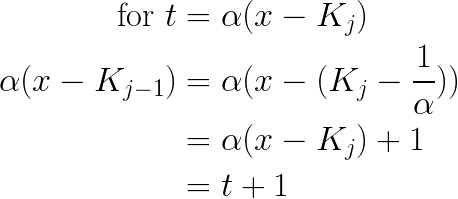We can apply this same manipulation to show that the arguments to B3, as centered around knot Kj, are simply {… t+2, t+1, t, t-1, t-2 …}.

By the definition of B3 above, you can see that B3(t) is non-zero only for t in [0,4), and so the four corresponding knot points Kj-3 … Kj contribute to its value: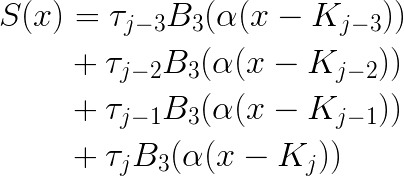This suggests a way to manipulate the equations into a standard form. In the transformed space t, the four nonzero terms are: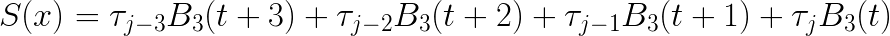and by plugging in the appropriate Ni for each term, we arrive at: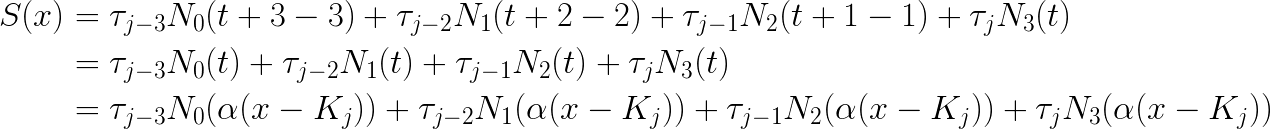Now, PolynomialSplineFunction is going to automatically identify the appropriate Kj and subtract it, and so I can define that transform as u = x - Kj, which gives: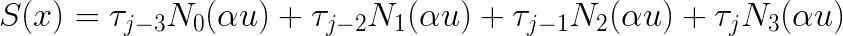I substitute the argument (αu) into the definitions of the four Ni to obtain: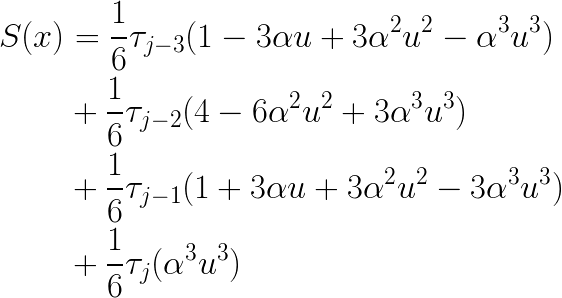Lastly, collecting like terms gives me the standard-form coefficients that I need for PolynomialSplineFunction: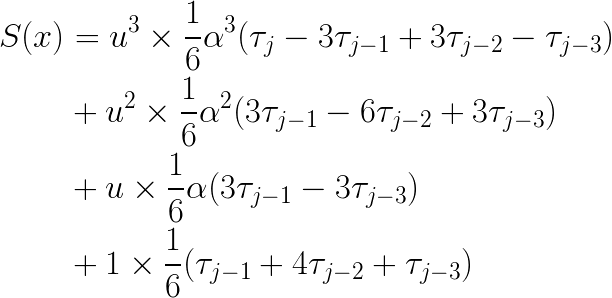Now I am equipped to return a PolynomialSplineFunction to my users, which implements the cubic B-spline that I fit to their data. Happy computing!

#### References

 H. Fujioka and H. Kano: Monotone smoothing spline curves using normalized uniform cubic B-splines, Trans. Institute of Systems, Control and Information Engineers, Vol. 26, No. 11, pp. 389–397, 2013